Question

Constant Acceleration Kinematics: Car A is traveling at 22.0 m/s and car B at 29.0 m/s. Car A is 300 m behind car B when the driver of car A accelerates his car with a uniform forward acceleration of 2.40 m/s2. How long after car A begins to accelerate does it take car A to overtake car B?

1.The taken is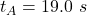Explanation:

Frm the question we are told that

The speed of car A isThe speed of car B is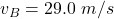The distance of car B  from A is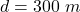The acceleration of car A is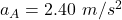For A to overtake B

The distance traveled by car B  =  The distance traveled by car A – 300m

Now the this distance traveled by car B before it is overtaken by A is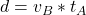Where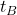is the time taken by car B

Now this can also be represented as using equation of motion as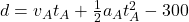Now substituting values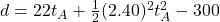Equating the both d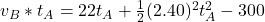substituting values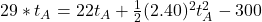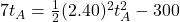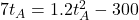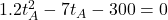Solving this using quadratic formula we have that